# Visualizing training with TensorBoard

• Last Updated : 05 Jul, 2021

In machine learning, to improve something you often need to be able to measure it. TensorBoard is a tool for providing the measurements and visualizations needed during the machine learning workflow. It enables tracking experiment metrics like loss and accuracy, visualizing the model graph, projecting NLP embeddings to a lower-dimensional space, and much more.

TensorBoard provides the following functionalities:

• Visualizing different metrics such as loss, accuracy with the help of different plots, and histograms.
• Visualize model layers and operations with the help of graphs.
• Provide histograms for weights and biases involved in training.
• Displaying training data (image, audio, and text data).

TensorBoard has the following tabs:

• Scalars: This tab is used to visualize the scalar metrics such as loss and accuracy.
• Graph: Visualize the computational graph of your models, such as the neural network model in the form layers and operations.
• Distributions: Visualize the training progression over time such as weight/bias changes.
• Histogram: Visualize the above distribution in the form of 3D-histograms.
• Projectors: This tab is used to visualize the word embedding for Natural Language Processing.
• Images: This tab is used to visualize the contents of training/test images data.
• Audio: This tab is used to visualize the audio data for application such as audio processing
• Text: This tab is used to visualize the audio data.

Implementation :

Code:

## python3

 `# Install it using pip` `!pip install ``-``q tf``-``nightly``-``2.0``-``preview`   `# To load tensorflow extension` `import` `tensorflow as tf` `import` `datetime, os` `# location of log directory` `logs_base_dir ``=` `"./logs"` `os.makedirs(logs_base_dir, exist_ok``=``True``)` `%``tensorboard ``-``-``logdir {logs_base_dir}`

• Plot Training Images:

Code:

## python3

 `# Import necessary modules` `import` `numpy as np` `import` `matplotlib.pyplot as plt` `import` `io`   `# Copy previous logs if any` `!rm ``-``rf .``/``logs``/`   `# Load datasets (Here,we use cifar 10` `cifar_10 ``=` `tf.keras.datasets.cifar10` `(x_train, y_train), (x_test,y_test) ``=` `cifar_10.load_data()`   `# List class Names` `class_names ``=``[``"airplane"``,``"automobile"``,``"bird"``,``"cat"``,``"deer"``,` `              ``"dog"``,``"frog"``,``"horse"``, ``"ship"``,``"truck"``]`   `# Data Preprocessing` `x_train ``=` `x_train.astype(``'float32'``)` `x_test ``=` `x_test.astype(``'float32'``)`   `x_train ``=` `x_train``/``255.0` `x_test ``=` `x_test``/``255.0`   `y_train  ``=` `tf.keras.utils.to_categorical(y_train)` `y_test  ``=` `tf.keras.utils.to_categorical(y_test)`   `# Creates a directory inside log/train_data folder` `# In which we store training images` `logdir ``=` `"logs/train_data/"` `+` `datetime.now().strftime(``"%Y%m%d-%H%M%S"``)` `# Creates a file writer for the log directory.` `file_writer ``=` `tf.summary.create_file_writer(logdir)`   `# write first 25 training images` `with file_writer.as_default():` `  ``# Reshape the images because tf.summary expects a 4 dimensional matrices` `  ``# having (batch_size,height, width, color_channels)` `  ``images ``=` `np.reshape(x_train[``0``:``25``], (``-``1``, ``32``, ``32``, ``3``))` `  ``tf.summary.image(``"Display training data"``, images, max_outputs``=``25``, step``=``0``)`   `# start TensorBoard and display those images (in images tab)` `%``tensorboard ``-``-``logdir logs``/``train_data`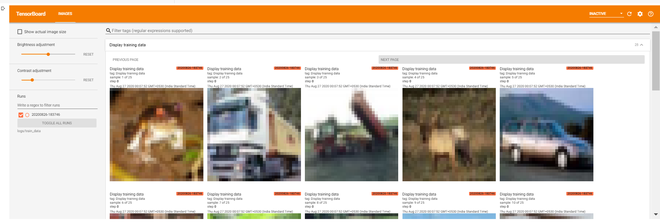Training Images

• Plot Images Data Using Matplotlib: We can see that the above training images are not clear. That’s because the above training images are of size (32, 32, 3) which is of very low resolution. Let’s plot some images in matplotlib.

Code:

## python3

 `# remove old plots data (if any)` `!rm ``-``rf logs``/``plots`   `logdir ``=` `"logs/plots/"` `+` `datetime.now().strftime(``"%Y%m%d-%H%M%S"``)` `file_writer ``=` `tf.summary.create_file_writer(logdir)`   `def` `plot_to_image(figure):` `  ``"""Converts the matplotlib plot to a PNG image and returns it.` `  ``The supplied figure is closed and inaccessible after this call."""` `  ``# Save the plot to a PNG in memory.` `  ``buf ``=` `io.BytesIO()` `  ``plt.savefig(buf, ``format``=``'png'``)` `  ``# Closing the figure prevents it from being displayed directly inside` `  ``# the notebook.` `  ``plt.close(figure)` `  ``buf.seek(``0``)` `  ``# Convert PNG buffer to TF image` `  ``image ``=` `tf.image.decode_png(buf.getvalue(), channels``=``4``)` `  ``# Add the batch dimension` `  ``print``(image.shape)` `  ``image ``=` `tf.expand_dims(image, ``0``)` `  ``return` `image`   `def` `image_grid():` `  ``"""Return a 5x5 grid of the training images as a matplotlib figure."""` `  ``# Create a figure to contain the plot.` `  ``figure ``=` `plt.figure(figsize``=``(``10``,``10``))` `  ``for` `i ``in` `range``(``25``):` `    ``# create the next subplot with class name as its title` `    ``plt.subplot(``5``, ``5``, i ``+` `1``, title ``=` `class_names[np.``int``(np.where(y_train[i] ``=``=``1``)[``0``])])` `    ``plt.xticks([])` `    ``plt.yticks([])` `    ``plt.grid(``False``)` `    ``plt.imshow(x_train[i])` `  `  `  ``return` `figure`   `# Prepare the plot` `figure ``=` `image_grid()` `# Convert to image and log` `with file_writer.as_default():` `  ``tf.summary.image(``"Training data"``, plot_to_image(figure), step``=``0``)` `# start tensorboard and display plot` `%``tensorboard ``-``-``logdir logs``/``plots`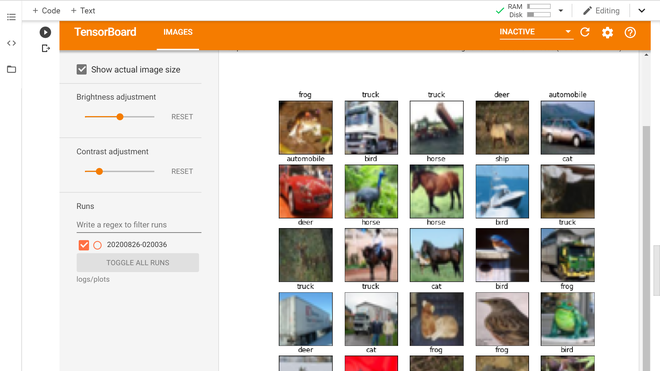Training Images using matplotlib

• Display Training Results Metrics: In this section, we will be plotting results metrics on TensorBoard. We will be using scalars and images tabs to display our results. For that, we will define a Convolutional Neural Network model and train it on CIFAR 10 dataset for 20 epoch.

Code:

## python3

 `# Define CNN model` `model  ``=` `tf.keras.models.Sequential([` `    ``tf.keras.layers.Conv2D(``32``, (``3``, ``3``), activation``=``'relu'``, padding``=``'same'``, input_shape``=``(``32``, ``32``, ``3``)),` `    ``tf.keras.layers.Conv2D(``32``, (``3``, ``3``), activation``=``'relu'``, padding``=``'same'``),` `    ``tf.keras.layers.MaxPooling2D((``2``, ``2``)),` `    ``tf.keras.layers.Dropout(``0.2``),` `    ``tf.keras.layers.Conv2D(``64``, (``3``, ``3``), activation``=``'relu'``, padding``=``'same'``),` `    ``tf.keras.layers.Conv2D(``64``, (``3``, ``3``), activation``=``'relu'``, padding``=``'same'``),` `    ``tf.keras.layers.MaxPooling2D((``2``, ``2``)),` `    ``tf.keras.layers.Dropout(``0.2``),` `    ``tf.keras.layers.Flatten(),` `    ``tf.keras.layers.Dense(``64``, activation``=``'relu'``),` `    ``tf.keras.layers.Dense(``10``, activation``=``'softmax'``)` `])`   `# Compile CNN model` `model.``compile``(` `    ``optimizer``=``tf.keras.optimizers.SGD(learning_rate``=` `0.01` `, momentum``=``0.1``), ` `    ``loss``=``'categorical_crossentropy'``,` `    ``metrics``=``[``'accuracy'``]` `)` `# Print model summary()` `model.summary()`

```Model: "sequential"
_________________________________________________________________
Layer (type)                 Output Shape              Param #
=================================================================
conv2d (Conv2D)              (None, 32, 32, 32)        896
_________________________________________________________________
conv2d_1 (Conv2D)            (None, 32, 32, 32)        9248
_________________________________________________________________
max_pooling2d (MaxPooling2D) (None, 16, 16, 32)        0
_________________________________________________________________
dropout (Dropout)            (None, 16, 16, 32)        0
_________________________________________________________________
conv2d_2 (Conv2D)            (None, 16, 16, 64)        18496
_________________________________________________________________
conv2d_3 (Conv2D)            (None, 16, 16, 64)        36928
_________________________________________________________________
max_pooling2d_1 (MaxPooling2 (None, 8, 8, 64)          0
_________________________________________________________________
dropout_1 (Dropout)          (None, 8, 8, 64)          0
_________________________________________________________________
flatten (Flatten)            (None, 4096)              0
_________________________________________________________________
dense (Dense)                (None, 64)                262208
_________________________________________________________________
dense_1 (Dense)              (None, 10)                650
=================================================================
Total params: 328,426
Trainable params: 328,426
Non-trainable params: 0
_________________________________________________________________```
• Now, we define the function to plot the confusion matrix using test data

Code:

## python3

 `# Code to plot confusion matrix` `def` `plot_confusion_matrix(cm, class_names):` `  ``"""` `  ``Returns a matplotlib figure containing the plotted confusion matrix.`   `  ``Args:` `    ``cm (array, shape = [n, n]): a confusion matrix of integer classes` `    ``class_names (array, shape = [n]): String names of the integer classes` `  ``"""` `  ``figure ``=` `plt.figure(figsize``=``(``8``, ``8``))` `  ``plt.imshow(cm, interpolation``=``'nearest'``, cmap``=``plt.cm.Blues)` `  ``plt.title(``"Confusion matrix"``)` `  ``plt.colorbar()` `  ``tick_marks ``=` `np.arange(``len``(class_names))` `  ``plt.xticks(tick_marks, class_names, rotation``=``45``)` `  ``plt.yticks(tick_marks, class_names)`   `  ``# Normalize the confusion matrix.` `  ``cm ``=` `np.around(cm.astype(``'float'``) ``/` `cm.``sum``(axis``=``1``)[:, np.newaxis], decimals``=``2``)`   `  ``# Use white text if squares are dark; otherwise black.` `  ``threshold ``=` `cm.``max``() ``/` `2.` `  ``for` `i, j ``in` `itertools.product(``range``(cm.shape[``0``]), ``range``(cm.shape[``1``])):` `    ``color ``=` `"white"` `if` `cm[i, j] > threshold ``else` `"black"` `    ``plt.text(j, i, cm[i, j], horizontalalignment``=``"center"``, color``=``color)`   `  ``plt.tight_layout()` `  ``plt.ylabel(``'True label'``)` `  ``plt.xlabel(``'Predicted label'``)` `  ``return` `figure`

• Now, we define the TensorBoard callback to display the confusion matrix of the model predictions over test data.

Code:

## python3

 `logdir ``=` `"logs/image/"` `+` `datetime.now().strftime(``"%Y%m%d-%H%M%S"``)`   `# Define the basic TensorBoard callback.` `tensorboard_callback ``=` `tf.keras.callbacks.TensorBoard(log_dir``=``logdir)` `# Create file Writer for Confusion Metrics` `file_writer_cm ``=` `tf.summary.create_file_writer(logdir ``+` `'/cm'``)`

• Now, we define the function to log the confusion matrix into the Tensorboard.

Code:

## python3

 `# sklearn confusion metrics` `from` `sklearn.metrics ``import` `confusion_matrix` `import` `itertools` `def` `log_confusion_matrix(epoch, logs):` `  ``# Use the model to predict the values from the validation dataset.` `  ``test_pred_raw ``=` `model.predict(x_test)` `  ``test_pred ``=` `np.argmax(test_pred_raw, axis``=``1``)` `  ``y_test_cls ``=` `np.argmax(y_test, axis``=``1``)`   `  ``# Calculate the confusion matrix.` `  ``cm ``=` `confusion_matrix(y_test_cls, test_pred)`   `  ``figure ``=` `plot_confusion_matrix(cm, class_names``=``class_names)` `  ``cm_image ``=` `plot_to_image(figure)`   `  ``# Log the confusion matrix as an image summary.` `  ``with file_writer_cm.as_default():` `    ``tf.summary.image(``"Confusion Matrix"``, cm_image, step``=``epoch)`   `# Define the per-epoch callback to plot confusion metrics after each epoch.` `cm_callback ``=` `tf.keras.callbacks.LambdaCallback(on_epoch_end``=``log_confusion_matrix)`

Code:

## python3

 `%``tensorboard ``-``-``logdir logs``/``image` `# Train the classifier.` `model.fit(` `    ``x_train,` `    ``y_train,` `    ``epochs``=``20``,` `    ``callbacks``=``[tensorboard_callback, cm_callback],` `    ``validation_data``=``(x_test, y_test)` `)`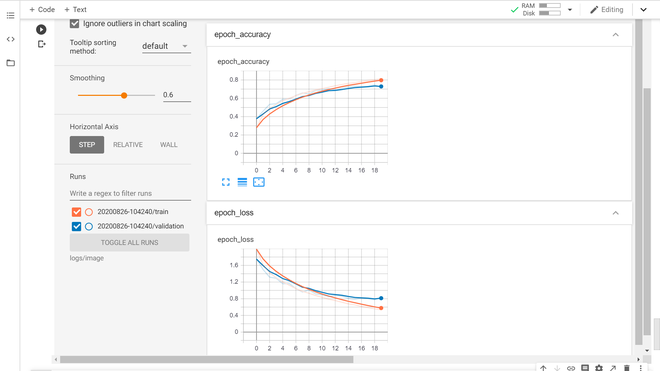Loss & Accuracy Graph (Scalar Tab)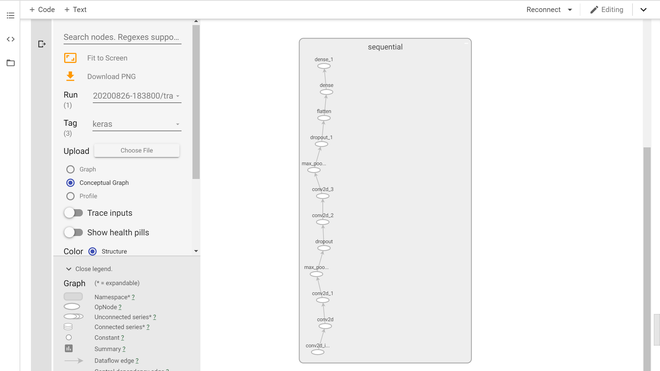Keras Model Graph (Graph Tab)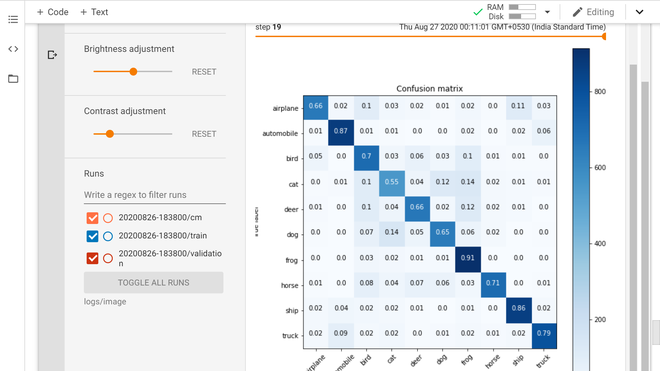Confusion Matrix (Images Tab)

References:

My Personal Notes arrow_drop_up
Recommended Articles
Page :